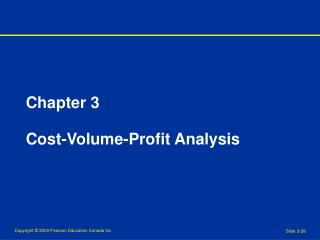DownloadDownload PresentationChapter 3 Cost-Volume-Profit Analysis

# Chapter 3 Cost-Volume-Profit Analysis

Download Presentation## Chapter 3 Cost-Volume-Profit Analysis

- - - - - - - - - - - - - - - - - - - - - - - - - - - E N D - - - - - - - - - - - - - - - - - - - - - - - - - - -
##### Presentation Transcript

1. Chapter 3Cost-Volume-Profit Analysis

2. Cost-Volume-Profit Analysis • Examines the behaviour of total revenues, total costs, and operating income as changes occur in the output level, selling price, variable costs, or fixed costs Assumptions of CVP Analysis • Revenues change in relation to production and sales • Costs can be divided in variable and fixed categories • Revenues and costs behave in a linear fashion • Costs and prices are known • If more than one product exists, the sales mix is constant • We can ignore the time value of money Page 67

3. Total for Per Unit 2 units % Revenue \$200 \$400 100% Variable costs 12024060% Contribution margin \$80 \$160 40% Contribution Margin • Contribution margin is equal to the difference between total revenue and total variable costs Contribution margin per unit = Selling price – Variable cost per unit Contribution margin percentage = Contribution margin per unit / Selling price per unit Pages 68 - 69

4. Contribution Margin Income Statement • Income statement that groups line items by cost behaviour to highlight the contribution margin Packages Sold 0 1 2 25 40 Revenue \$0 \$200 \$400 \$5,000 \$8,000 Variable costs 0 120 240 3,000 4,800 Contribution margin 0 80 160 2,000 3,200 Fixed costs 2,000 2,000 2,000 2,000 2,000 Operating income \$(2,000) \$(1,920) \$(1,840) \$0 \$1,200 Page 69

5. Breakeven Point • Quantity of output where total revenues equal total costs • Point where operating income equals zero Breakeven point in units = Fixed costs / Contribution margin per unit = \$2,000 / \$80 = 25 units Breakeven point in dollars = Fixed costs / Contribution margin % = \$2,000 / 40% = \$5,000 Page 71

6. Cost-Volume-Profit Graph \$10,000 \$8,000 \$6,000 \$4,000 \$2,000 \$0 Total revenues line Breakeven Point 25 units Total costs line Operating income Operating loss 0 10 20 30 40 50 Units Sold Page 72

7. Target Operating Income • For most firms in the private sector, the main objective is not to break even • Convert after-tax desired net income to its before-tax equivalent operating income Target operating income = Target net income / (1 - tax rate) Target Unit Sales = (Fixed costs + Target operating income) / Contribution margin per unit Target Dollar Sales = (Fixed costs + Target operating income) / Contribution margin % Pages 73 - 75

8. Sensitivity Analysis • Sensitivity analysis is a “what-if” technique that examines how a result will change if the original predicted data are not achieved or if an underlying assumption changes • What will happen to operating income if volume declines by 5%? • What will happen to operating income if variable costs increase by 10% per unit? • Sensitivity analysis broadens management’s perspectives about possible outcomes Pages 76 - 77

9. Option 2 \$1,400 Fixed Fee + 5% Commission Option 1 \$2,000 Fixed Fee Option 3 20% Commission Rev Rev Rev \$ \$ \$ Cost Cost Cost Units Units Units Breakeven = 25 units Breakeven = 20 units Breakeven = 0 units Alternative Cost Structures • CVP helps managers assess the risks and potential benefits of adopting alternative cost structures Example: Alternative rental arrangements Pages 77 - 78

10. Revenue Mix • Revenue mix (or sales mix) is the relative combination of quantities of products or services that make up total revenue • Sales mix of Do-All : Superword = 2 : 1 Breakeven point in units = 30 units 20 units of Do-All 10 units of Superword Do-All Do-All Superword Pages 73 - 75

11. Multiple Cost Drivers • In many cases there may be multiple cost drivers Do-All Software Example Variable costs: \$40 per software package sold \$15 per invoice issued Operating income = Revenue – (\$40 x Packages sold) – (\$15 x Invoices issued) – Fixed costs • In cases where there are multiple cost drivers there are multiple breakeven points Pages 81 - 82

12. Contribution Margin & Gross Margin Merchandising Sector Contribution Margin Format Revenues \$200 Variable costs: Cost of goods sold \$120 Other variable 43 163 Contribution margin 37 Fixed costs: Cost of goods sold 5 Other fixed 19 24 Operating income \$13 Gross Margin Format Revenues \$200 Cost of goods sold (120+5) 125 Gross margin 75 Operating costs (43+19) 62 Operating income \$13 Pages 82 - 83

13. Contribution Margin & Gross Margin Manufacturing Sector Contribution Margin Format Revenues \$1,000 Variable costs: Manufacturing \$250 Non-manufacturing 270 520 Contribution margin 480 Fixed costs: Manufacturing 160 Non-manufacturing 138 298 Operating income \$182 Gross Margin Format Revenues \$1,000 Cost of goods sold (250+160) 410 Gross margin 590 Non-manufacturing (270+138) 408 Operating income \$182 Pages 81 - 82

14. Decision Models and Uncertainty • Managers make predictions and decisions in a world of uncertainty • Estimate events that are likely to occur and assign probabilities to each outcome • Probability distribution describes the likelihood of each mutually exclusive and collectively exhaustive set of events (must add to 1.00) • Expected value is a weighted average of the outcomes with the probability of each outcome serving as the weight Pages 86 - 87

15. Uncertainty Example Proposal A: Spy Novel 0.5 Probability 0.4 0.3 0.2 0.1 • 2 3 4 5 6 7 8 9 Cash Inflow (\$000,000) Expected value = (0.1*\$300,000) + (.02*\$350,000) + (.04*\$400,000) + (0.2*\$450,000) + (0.1*\$500,000) = \$400,000 Page 87